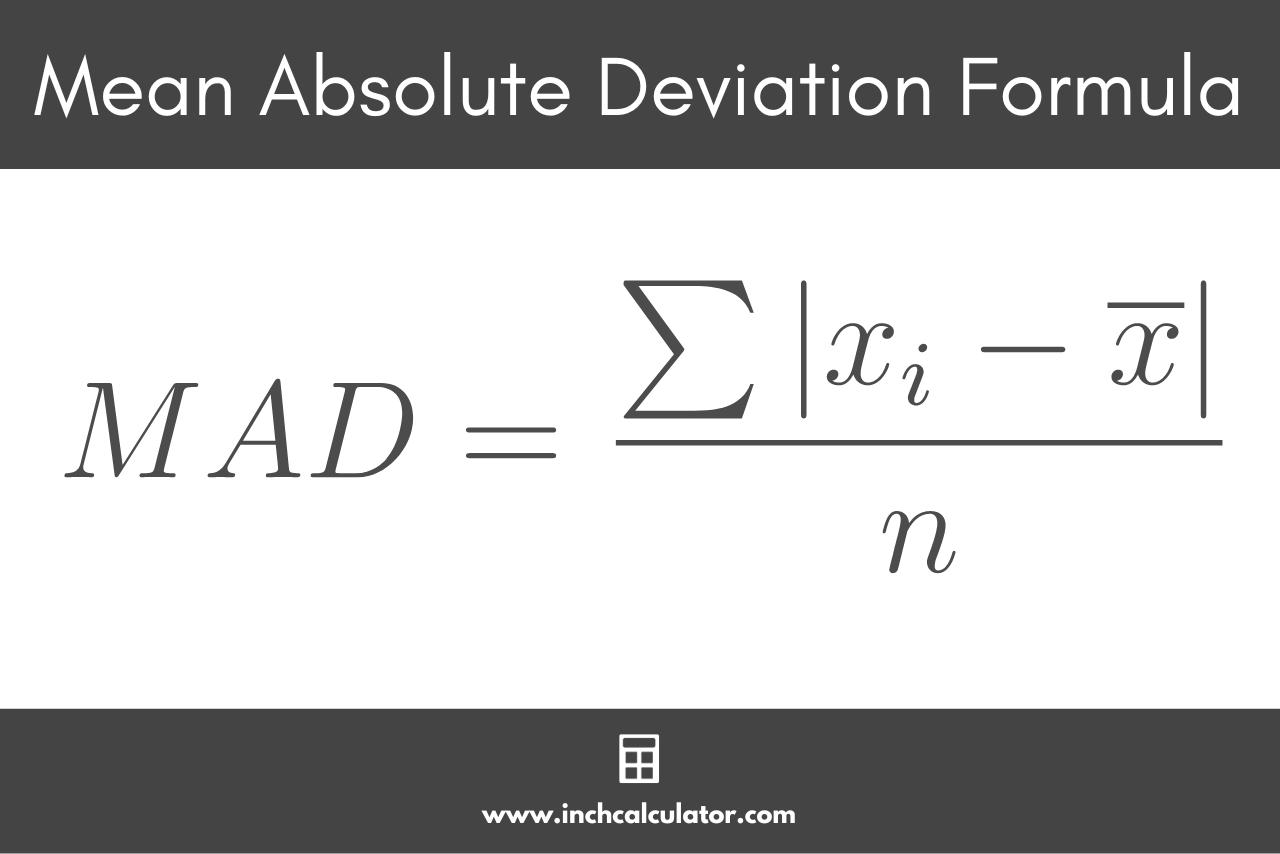# Mean Absolute Deviation Calculator – Find MAD

Find the mean absolute deviation of a data set by entering the numbers below.

Separate numbers using a comma (,)

## Mean Absolute Deviation:

### Steps to Solve

#### Step One: Find the Mean

\bar{x} = \frac{\sum x_{i}}{n}

#### Step Two: Find the Absolute Value of the Difference (Δ) Between Each Number and the Mean

\Delta = |x_{i}-\bar{x}|

#### Step Three: Find the MAD

Learn how we calculated this below

## How to Find the Mean Absolute Deviation

In statistics, the mean absolute deviation, or MAD, is the average difference between each value in the set and the mean.

Like standard deviation, mean absolute deviation is a measure of the variability in a data set. While standard deviation is the square root of the sum of squares of the deviations from the mean, MAD is the mean of those deviations.

### Mean Absolute Deviation Formula

You can calculate the mean absolute deviation using a simple formula:

Where:
xi = each number in the set
= the mean
n = size of the set

So, the mean absolute deviation MAD is equal to the sum of the differences between each number in the set xi and the mean , divided by the size of the set nYou can use the formula above to find the mean absolute deviation in a few easy steps.

### Step One: Calculate the Mean

In order to use the formula above to calculate the MAD, you’ll first need to find the mean of the data. You can find the mean by adding each value in the set, then dividing by the size of the set.

x̄ = ∑xi ÷ n

### Step Two: Calculate the Difference Between Each Value

Next, find the absolute value of the difference between each number and the mean. Subtract the mean from each number in the set and find the absolute value.

### Step Three: Calculate the Mean Absolute Deviation

Finally, put it all together to find the mean absolute deviation. Sum up all of the differences together, then divide that by the number of elements in the set.

For example: let’s find the mean absolute deviation for the numbers [2,7,15,22]

Let’s start by finding the mean.

x̄ = (2 + 7 + 15 + 22) ÷ 4
x̄ = 46 ÷ 4
x̄ = 11.5

Next, let’s find the absolute value of the difference between each number and the mean.

|2 – 11.5| = 9.5
|7 – 11.5| = 4.5
|15 – 11.5| = 3.5
|22 – 11.5| = 10.5

Then, sum the differences together.

sum = 9.5 + 4.5 + 3.5 + 10.5
sum = 28

Finally, divide by the number of values in the set, which is 4.